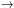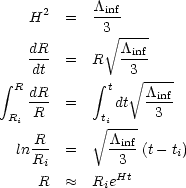3.1. Friedmann's EquationExponential Expansion

One way to describe inflation is that during inflation, ainf term dominates Eq. 11. Thus, during inflation we have,(22) (23) (24) (25) (26)

where ti and Ri are the time and scale factor at the beginning of inflation. To get Eq. 26 we have assumed 0ti << t < te (where te is the end of inflation) and we have used Eq. 22. Equation 26 is the exponential expansion of the Universe during inflation. The e-folding time is 1/H. The doubling time is (ln 2) / H. That is, during every intervalt = 1/H, the size of the Universe increases by a factor of e = 2.718281828... and during every intervalt = (ln 2) / H the size of the Universe doubles.